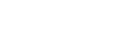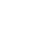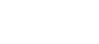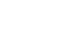## Mathematics Paper 1 Questions and Answers - Lanjet Joint Mock Exams 2022

INSTRUCTION TO CANDIDATE’S:

1. Write your name, index number and school in the spaces provided at the top of this page.
2. Sign and write the date of examination in spaces provided above.
3. This paper consists of TWO sections: Section I and Section II.
4. Answer ALL the questions in Section I and any five questions from Section II.
5. Show all the steps in your calculation, giving your answer at each stage in the spaces provided below each question.
6. Marks may be given for correct working even if the answer is wrong.
7. Non-programmable silent electronic calculators and KNEC Mathematical tables may be used, except where stated otherwise.
8. Candidates should answer the questions in English.

### QUESTIONS

SECTION I: (50 MARKS)
Answer all the questions in this section in the spaces provided.

1. Two signals have been set to flash at interval of 15 minutes, 24 minutes if they flash at 8.13am. When will they flash together again? (2 marks)
2. Solve for m in the equation: (3 marks)
34(m + 1) + 34m = 246
3. Use tables of cubes, cube roots and reciprocals to find the value of;4. Evaluate: (3 marks)5. If tan X = 4/3, find the value of sin2X + cos X without using tables or calculator. (3marks)
6. Triangle A1B1C1 is the image of triangle ABC under the transformation represented by the matrix.If the area of triangle A1B1C1 is 140cm2, find the area of triangle ABC. (3 marks)
7. Use the exchange rates below to answer this question
 Buying selling 1 US dollar 63.00 63.20 1 UK£ 125.30 125.95
A tourist arriving in Kenya from Britain had 9600 UK sterling pounds (£). He converted the pounds to Kenya shillings at a commission of 5%. While in Kenya he spent ¾ of this money. He changed the balance to US dollars after his stay. If he was not charged any commission for this last transaction, calculate to the
nearest US dollars the amount he received. (3mks)
8. Convert 0.123into a fraction. (3 marks)
9. A train moving at an average speed of 72km/hr. takes 15 seconds to complete cross a bridge that is 80m long.Find the length of the train. (3 marks)
10. The interior angle of a regular polygon is 6½ times the exterior angle. How many sides does the polygon have? (3 marks)
11. Simplify:(3 marks)
12. A solid metal cuboid 1.5m long, 0.4m wide and 0.25m high is made of material of density 7.5g/cm³. Calculate its mass in kg. (3 marks)
13. Find the equation of a straight line which is equidistant from the points A (2, 3) and B (6, 1). Express your answer in the formwhere a and b are constant. (3marks)
14. Ruto is 21/4 times as old as his son. Five years ago, the ratio of their ages was 8:3. What will be their ages 6 years from now? (4 marks)
15. Two similar cylinders have diameter of 7cm and 21cm. If the larger cylinder has a mass of 6237g. Find the mass of the smaller cylinders. (4 marks)
16. Find the inequalities that define the region R shown in the figure below. (3 marks)SECTION B: (50 MARKS)
Answer any FIVE questions from this section.

1. Samatha and Meshi entered into a business partnership in which they contributed Kshs. 120,000 and Kshs. 150,000 every year respectively. After one year Fuki joined the business and contributed Kshs.90,000
1. Calculate the ratio of their investment after 3 years of business (3mks)
2. It was agreed that 30% of the profits after 3 years be used to cater for the cost of running the business, while the remaining would be shared proportionally. Calculate each person’s share if the profit made after three years was Kshs, 187,000. (4mks)
3. If each of them invested their shares in the business, find their new individual investments at the beginning of the fourth year. (3mks)
2. In the figure below OQ = q and OR = r. Point X divides OQ in the ratio 1:2 and Y divides OR in the ratio 3:4. Lines XR and YQ intersect at E.1. Express in terms of q and r.
1. XR. (1 mark)
2. YQ. (1 mark)
2. If XE = mXR and YE = nYQ, express OE in terms of
1. r, q and m. (1 mark)
2. r, q and n. (1 mark)
3. Using the results in (b) above, find the values of m and n. (6 marks)
3. A car accelerates from rest for 10seconds until it reaches a velocity of 12meters per second. It then continues at this velocity for the next 40 seconds after which it breaks are applied and it comes to rest at a constant retardation of 1.5meters per second squared.
1. Determine
1. The acceleration over the first 10seconds (2mks)
2. The time taken during the retardation (2mks)
2. Draw the velocity time graph for the journey and use it to determine
1. The total distance covered by the car (4mks)
2. The percentage of the total distance which was covered during the first 15 seconds. (2mks)
4.
1. Complete the table given below for the equation y = -2x² + 3x + 3 for the range -2 ≤ x ≤ 3.5 by filling in the blank spaces. (2 marks)
 x -2 -1.5 -1 -0.5 1 1.5 2 2.5 3 3.5 y -6 1 -2 -11
2. Use the values from the table above to draw the graph of y = -2x² + 3x + 3. (3 marks)
1. determine the integral values of x in the graphs range which satisfy the inequality
2x² - 3x - 3 ≥ 3. (3 marks)
2. Solve -2x² + 2x + 5 = 0. (2 marks)
5. A sector of a circle of radius 40cm subtends an angle of 26º at the centre of the circle.
Take π = 22/7
1. Calculate
1. The area of the sector. (2 marks)
2. The length of the arc. (2 marks)
2. The sector is folded to form an inverted right cone. Calculate
1. The base radius of the cone. (2 marks)
2. To one decimal place, the vertical height of the cone. (2 marks)
3. Calculate the capacity of the cone in litres. (2 marks)
6. The table below shows marks obtained by 100 candidates at Eastside High School in a Biology examination.
 marks 15-24 25-34 35-44 45-54 55-64 65-74 75-84 85-94 frequency 6 14 24 14 x 10 6 4
1. Determine the value of x. (2 marks)
2. State the modal frequency. (1 mark)
3. Calculate the median mark. (4 marks)
4. Calculate the mean mark. (3 marks)
7. A straight line passes through points (8, -2) and (4, -4)
1. Write its equation in the form ax + by + c = 0 where a, b and c are integers. (3 marks)
2. If the line in (a) above cuts the x axis at point P, determine the coordinates of P. (2 marks)
3. Another line which is perpendicular to the line in (a) above passes through point P and cuts the Y axis at the point Q. Determine the co-ordinates of point Q. (3 marks)
4. Find the length of QP. (2 marks)
8.
1. After t seconds, a particle moving along a straight line has a velocity of V m/s and an acceleration of (5 – 2t) m/s². The particles initial velocity is 2m/s.
1. Express V in terms of t. (3 marks)
2. Determine the velocity of the particle at the beginning of the third second. (2 marks)
2. Find the time taken by the particle to attain maximum velocity and the distance it covered to attain the maximum velocity. (5 marks)

### MARKING SCHEME

SECTION I: (50 MARKS)
Answer all the questions in this section in the spaces provided.

1. Two signals have been set to flash at interval of 15 minutes, 24 minutes if they flash at 8.13am. When will they flash together again? (2 marks)
2   15   24
2   15   12
2   15   6
3   15   3
5   5   1
1    1
l.c.m = 8 x 15 = 120 mins
120/60 = 2 hrs
8:13 + 2:00 = 10:13 am
2. Solve for m in the equation: (3 marks)
34(m + 1) + 34m = 246
34m x 34 + 34m = 246
let 34m - p
81p + p = 246
82p = 246
p = 3
34m = 31
4m = 1
3. Use tables of cubes, cube roots and reciprocals to find the value of;4(1/8.63 x 10)3
4(1/642.7 x 103)
4(0.1556 x 10-5)
4 x 0.0001556
0.6223
(5/3446 x 101)1/3
(5 x 0.2902 x 10-8)1/3
(0.14510)1/3
(145.1 x 10-3)1/3
5.255 x 10-1
0.5255
0.6223 + 0.5255
1.1478
4. Evaluate: (3 marks)1/2 x 7/2 + 3/2(5/2 - 2/3)
5/2 - 2/3 = 15 - 4 = 11
6         6
3/2 x 11/6 = 23/4 = 11/4
1/2 x 7/2 = 7/4
7/4 + 11/4 = 18/4
3/4 x 5/2 x 2/1 = 33/4 = 15/4
18/4 x 4/15 = 6/5 = 11/5
5. If tan X = 4/3, find the value of sin2X + cos X without using tables or calculator. (3marks)sin2x = (4/5)2 = 16/25
cos x = 3/5
sin2x + cos x = 16/25 + 3/5
16 + 15 = 31 = 1  6
25       25       25
6. Triangle A1B1C1 is the image of triangle ABC under the transformation represented by the matrix.If the area of triangle A1B1C1 is 140cm2, find the area of triangle ABC. (3 marks)
A.S.F = IDetI
140/x = 12 - 5
7x = 140
x = 140/7
x = 20cm2
7. Use the exchange rates below to answer this question
 Buying selling 1 US dollar 63.00 63.20 1 UK£ 125.30 125.95
A tourist arriving in Kenya from Britain had 9600 UK sterling pounds (£). He converted the pounds to Kenya shillings at a commission of 5%. While in Kenya he spent ¾ of this money. He changed the balance to US dollars after his stay. If he was not charged any commission for this last transaction, calculate to the
nearest US dollars the amount he received. (3mks)
9600 x 125.30 x 0.95 = 1142736
1142736 x 1/4 = sh. 285.684
285684 = 4520.316
63.20
= 4520 us dollars
8. Convert 0.123into a fraction. (3 marks)
1000r = 123.123
r   =   0.123
999r  = 123
r = 123/999
r = 41/333
9. A train moving at an average speed of 72km/hr. takes 15 seconds to complete cross a bridge that is 80m long.Find the length of the train. (3 marks)
72 x 5/18 = 20 m/s
80 + x = 15
20
300 = 80 + x
x = 300 - 80
= 220 m
10. The interior angle of a regular polygon is 6½ times the exterior angle. How many sides does the polygon have? (3 marks)
x + 61/2x = 180
71/2 x = 180
x = 24
n = 360/24 = 15 sides
11. Simplify:(3 marks)
3a2 - 48 = 3(a + 4)(x - 4)
3a2 - 24a + 3a2 = 3(a2 - 8a + 16)
p = 16
s = -3
a2 - 4a - 4a + 16
a(a - 4) - 4(a - 4)
9a - 4)(a - 4)
D = 3(a - 4)(a - 4)
3(a - 4)(a + 4)
3(a - 4)(a - 4)
= a + 4
a - 4
12. A solid metal cuboid 1.5m long, 0.4m wide and 0.25m high is made of material of density 7.5g/cm³. Calculate its mass in kg. (3 marks)
v = 150 x 40 x 25 = 150,000 cm3
m = d x v
= 150000 x 7.5
= 1,125,000 g
1000
= 1125 kg
13. Find the equation of a straight line which is equidistant from the points A (2, 3) and B (6, 1). Express your answer in the formwhere a and b are constant. (3marks)
midpoint of AB = (2 + 63 + 1)
2          2
= (4, 2)
m1 = 1 - 3 = -2 = -1
6 - 2     4      2
m2 = -1  = 2
-1/2
2 = y - 2
x - 4
2x - 8 = y - 2
2x - y = 6
6    6    6
x + y = 1
3   -6
14. Ruto is 21/4 times as old as his son. Five years ago, the ratio of their ages was 8:3. What will be their ages 6 years from now? (4 marks)
now              5 yrs ago
ruto       21/4x            9/4x - 5
son         x                  x - 5
9/4x - 5 = 8
x - 5      3
27x - 15 = 8x - 40
25 = 5/4x
x = 20 yrs
son = 20 + 6 = 26 years
ruto (9/4 x 20) + 6 = 51 years
15. Two similar cylinders have diameter of 7cm and 21cm. If the larger cylinder has a mass of 6237g. Find the mass of the smaller cylinders. (4 marks)
l.s.f = 21/7 = 3/1
v.s.f = 27/1
27/1 = 6237/x
27x/27 = 6237/27
x = 231 g
16. Find the inequalities that define the region R shown in the figure below. (3 marks)L1 => x ≥ 0
L2 => x/5 + y/4 = 1
4x + 5y = 1
20
4x + 5y = 20
4x + 5y < 20
L3 => x/4 + y/-3 = 1
-3x + 4y = 1
-12
-3x + 4y = -12
3x - 4y = 12
3x - 4y ≤ 12

SECTION B: (50 MARKS)
Answer any FIVE questions from this section.

1. Samatha and Meshi entered into a business partnership in which they contributed Kshs. 120,000 and Kshs. 150,000 every year respectively. After one year Fuki joined the business and contributed Kshs.90,000
1. Calculate the ratio of their investment after 3 years of business (3mks)
S:M:F = 120,000 x 3: 150,000 x 3 : 90,000 x 2
= 360, 000 : 450, 000 : 180,000
= 4 : 5 : 2
2. It was agreed that 30% of the profits after 3 years be used to cater for the cost of running the business, while the remaining would be shared proportionally. Calculate each person’s share if the profit made after three years was Kshs, 187,000. (4mks)
70/100 x 187,000 = sh 130,900
samantha = 4/11 x 130900= sh 47600
meshi = 5/11 x 130900 = sh 59, 500
fuki = 2/11 x 130, 900 = sh 23800
3. If each of them invested their shares in the business, find their new individual investments at the beginning of the fourth year. (3mks)
samantha = 360000 + 130900 x 4/11 = sh. 407 600
meshi = 450,000 + 59 500 = sh 509,500
fuki = 180,000 + 223800 = sh 203800
2. In the figure below OQ = q and OR = r. Point X divides OQ in the ratio 1:2 and Y divides OR in the ratio 3:4. Lines XR and YQ intersect at E.1. Express in terms of q and r.
1. XR. (1 mark)
= r - 1/3q
2. YQ. (1 mark)
= q - 3/7r
2. If XE = mXR and YE = nYQ, express OE in terms of
1. r, q and m. (1 mark)
OE = OX + XE
= 1/3 t + -1/3qm + mr
OE = 1/3t(1 - m) + mI
2. r, q and n. (1 mark)
ans = 3/7r(1 - n) + nq
3. Using the results in (b) above, find the values of m and n. (6 marks)
3/7r (1 - n) = mr
3/7 - 3/7n = m
nq = 1/3q (1 - m)
n = 1/3 - 1/3m
n = 1/3 - 1/3 (3/7 - 3/7n)
n = 1/3 - 1/7 + 1/7n
6/7n = 4/21
n = 4/21 x 7/6 = 2/9
m = 3/7 - 3/7(2/9)
m =1/3
3. A car accelerates from rest for 10seconds until it reaches a velocity of 12meters per second. It then continues at this velocity for the next 40 seconds after which it breaks are applied and it comes to rest at a constant retardation of 1.5meters per second squared.
1. Determine
1. The acceleration over the first 10seconds (2mks)
12 - 0 = 1.2 m/s2
10
2. The time taken during the retardation (2mks)
-1.5 = 0 - 12
t
-1.5t = -12
t = -12 = 8 seconds
-1.5
2. Draw the velocity time graph for the journey and use it to determine
1. The total distance covered by the car (4mks)Distance  = 1/2 (58 + 40)12
= 588 m
2. The percentage of the total distance which was covered during the first 15 seconds. (2mks)
Distance = 1/2(15 + 5) 12
= 120m
120/588 x 100%
= 20.41%
4.
1. Complete the table given below for the equation y = -2x² + 3x + 3 for the range -2 ≤ x ≤ 3.5 by filling in the blank spaces. (2 marks)
 x -2 -1.5 -1 -0.5 0 0.5 1 1.5 2 2.5 3 3.5 y -11 -6 -2 1 3 4 4 3 1 -2 -6 -11
2. Use the values from the table above to draw the graph of y = -2x² + 3x + 3. (3 marks)1. determine the integral values of x in the graphs range which satisfy the inequality
2x² - 3x - 3 ≥ 3. (3 marks)
y = -2x2 + 3x + 3
+ 0 = 2x2 - 3x - 6
y = 0 + 0 - 3
y = -3
y ≤ -3
Range = -1.2 ≤ x ≤ 2.8
2. Solve -2x² + 2x + 5 = 0. (2 marks)
y = -2x2 + 3x + 3
- 0 = -2x2 + 3x + 5
y = 0 + 0 - 2
y = -2
x = -1  or  x = 2.5
5. A sector of a circle of radius 40cm subtends an angle of 26º at the centre of the circle.
Take π = 22/7
1. Calculate
1. The area of the sector. (2 marks)
26/360 x 22/7 x 40 x 40 = 363.1746 cm2
2. The length of the arc. (2 marks)
26/360 x 22/7 x 2 x 40 = 18.1587 cm
2. The sector is folded to form an inverted right cone. Calculate
1. The base radius of the cone. (2 marks)
22/7 x 2 x r = 18.1587
r = 18.1587 x 7/22 x 1/2
r = 2.889 cm
2. To one decimal place, the vertical height of the cone. (2 marks)
h = √402 - 2.8892
h = 39.9 cm
3. Calculate the capacity of the cone in litres. (2 marks)
v = 1/3 x 22/7 x 2.8892 x 39.9 = 120.76cm3 x 2.889 = 348.85
= 120.76/1000 = 0.12076L x 2.889 = 0.34886
= 0.34886L
6. The table below shows marks obtained by 100 candidates at Eastside High School in a Biology examination.
 marks 15-24 25-34 35-44 45-54 55-64 65-74 75-84 85-94 frequency 6 14 24 14 x 10 6 4
1. Determine the value of x. (2 marks)
x = 100 - 78 = 22
2. State the modal frequency. (1 mark)
= 24
3. Calculate the median mark. (4 marks)
 marks f c.f x fx 15-24 6 6 19.5 117 25-34 14 20 29.5 413 35-44 24 44 39.5 948 45-54 14 58 49.5 693 55-64 22 80 59.5 1309 65-74 10 90 69.5 695 75-84 6 96 79.5 477 85-94 4 100 89.5 358 100 5010
1/2 x 100 = 50th
median = 44.5 + (50 - 44) 10
14
= 44.5 + 4.2857
= 48.786
4. Calculate the mean mark. (3 marks)
mean = 5010
100
= 50.1
7. A straight line passes through points (8, -2) and (4, -4)
1. Write its equation in the form ax + by + c = 0 where a, b and c are integers. (3 marks)
m = -4 - -2 = -2 = 1
4 - 8      -4     2
y - -2 = 1
x - 8     2
2y + 4 = x - 8
x - 2y - 12 = 0
2. If the line in (a) above cuts the x axis at point P, determine the coordinates of P. (2 marks)
x - 2y = 12
12 12    12
x/12 + y/-6 = 1
p(12,0)
3. Another line which is perpendicular to the line in (a) above passes through point P and cuts the Y axis at the point Q. Determine the co-ordinates of point Q. (3 marks)Q(6, -12)
4. Find the length of QP. (2 marks)8.
1. After t seconds, a particle moving along a straight line has a velocity of V m/s and an acceleration of (5 – 2t) m/s². The particles initial velocity is 2m/s.
1. Express V in terms of t. (3 marks)
v = (5 - 2t) dt
v = 5t - 2t2/2 + c
v = 5t - t2 + c
2 = 0 - 0 + c
c = 2
v = 5t - t2 + 2
2. Determine the velocity of the particle at the beginning of the third second. (2 marks)
v = 5(2) - (2)2 + 2
v = 10 - 4 + 2
v = 8m/s
2. Find the time taken by the particle to attain maximum velocity and the distance it covered to attain the maximum velocity. (5 marks)
for max v , dv/dt = o
5 - 2t = 0
2t = 5
t = 2.5 seconds
s = (5t - t2 + 2) dt
s = 5/2t2 - t3/3 + 2t/1 + c
when t = 0, s = 0
0 = 0 - 0 + 0 + c
s = 2.5t2 - 1/2t3 + 2t
s = 2.5(2.5)2 - 1/3(2.5)3 + 2(2.5)
s = 15.625 - 125/24 + 5
= 1510/24
= 15.416 m

• ✔ To read offline at any time.
• ✔ To Print at your convenience
• ✔ Share Easily with Friends / Students